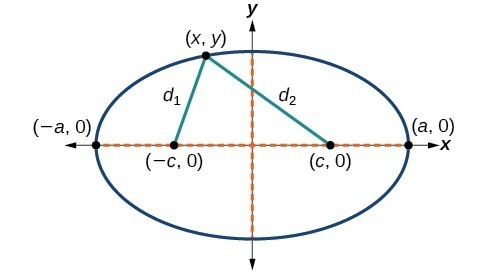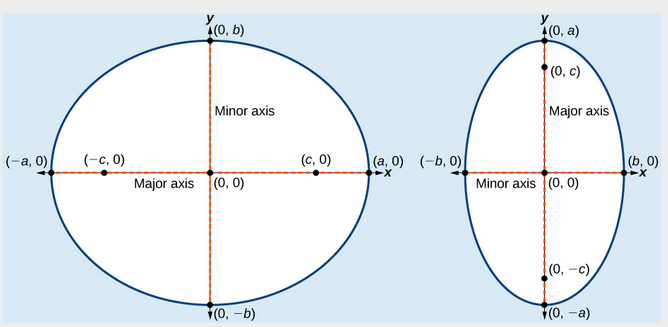## Deriving the Equation of an Ellipse Centered at the Origin

To derive the equation of an ellipse centered at the origin, we begin with the foci $\left(-c,0\right)$ and $\left(c,0\right)$. The ellipse is the set of all points $\left(x,y\right)$ such that the sum of the distances from $\left(x,y\right)$ to the foci is constant, as shown in Figure 5.Figure 5

If $\left(a,0\right)$ is a vertex of the ellipse, the distance from $\left(-c,0\right)$ to $\left(a,0\right)$ is $a-\left(-c\right)=a+c$. The distance from $\left(c,0\right)$ to $\left(a,0\right)$ is $a-c$ . The sum of the distances from the foci to the vertex is

$\left(a+c\right)+\left(a-c\right)=2a$

If $\left(x,y\right)$ is a point on the ellipse, then we can define the following variables:

$\begin{array}{l}{d}_{1}=\text{the distance from }\left(-c,0\right)\text{ to }\left(x,y\right)\hfill \\ {d}_{2}=\text{the distance from }\left(c,0\right)\text{ to }\left(x,y\right)\hfill \end{array}$

By the definition of an ellipse, ${d}_{1}+{d}_{2}$ is constant for any point $\left(x,y\right)$ on the ellipse. We know that the sum of these distances is $2a$ for the vertex $\left(a,0\right)$. It follows that ${d}_{1}+{d}_{2}=2a$ for any point on the ellipse. We will begin the derivation by applying the distance formula. The rest of the derivation is algebraic.

$\begin{array}{ll}\text{ }{d}_{1}+{d}_{2}=\sqrt{{\left(x-\left(-c\right)\right)}^{2}+{\left(y - 0\right)}^{2}}+\sqrt{{\left(x-c\right)}^{2}+{\left(y - 0\right)}^{2}}=2a\hfill & \text{Distance formula}\hfill \\ \sqrt{{\left(x+c\right)}^{2}+{y}^{2}}+\sqrt{{\left(x-c\right)}^{2}+{y}^{2}}=2a\hfill & \text{Simplify expressions}\text{.}\hfill \\ \text{ }\sqrt{{\left(x+c\right)}^{2}+{y}^{2}}=2a-\sqrt{{\left(x-c\right)}^{2}+{y}^{2}}\hfill & \text{Move radical to opposite side}\text{.}\hfill \\ \text{ }{\left(x+c\right)}^{2}+{y}^{2}={\left[2a-\sqrt{{\left(x-c\right)}^{2}+{y}^{2}}\right]}^{2}\hfill & \text{Square both sides}\text{.}\hfill \\ \text{ }{x}^{2}+2cx+{c}^{2}+{y}^{2}=4{a}^{2}-4a\sqrt{{\left(x-c\right)}^{2}+{y}^{2}}+{\left(x-c\right)}^{2}+{y}^{2}\hfill & \text{Expand the squares}\text{.}\hfill \\ \text{ }{x}^{2}+2cx+{c}^{2}+{y}^{2}=4{a}^{2}-4a\sqrt{{\left(x-c\right)}^{2}+{y}^{2}}+{x}^{2}-2cx+{c}^{2}+{y}^{2}\hfill & \text{Expand remaining squares}\text{.}\hfill \\ \text{ }2cx=4{a}^{2}-4a\sqrt{{\left(x-c\right)}^{2}+{y}^{2}}-2cx\hfill & \text{Combine like terms}\text{.}\hfill \\ \text{ }4cx - 4{a}^{2}=-4a\sqrt{{\left(x-c\right)}^{2}+{y}^{2}}\hfill & \text{Isolate the radical}\text{.}\hfill \\ \text{ }cx-{a}^{2}=-a\sqrt{{\left(x-c\right)}^{2}+{y}^{2}}\hfill & \text{Divide by 4}\text{.}\hfill \\ \text{ }{\left[cx-{a}^{2}\right]}^{2}={a}^{2}{\left[\sqrt{{\left(x-c\right)}^{2}+{y}^{2}}\right]}^{2}\hfill & \text{Square both sides}\text{.}\hfill \\ \text{ }{c}^{2}{x}^{2}-2{a}^{2}cx+{a}^{4}={a}^{2}\left({x}^{2}-2cx+{c}^{2}+{y}^{2}\right)\hfill & \text{Expand the squares}\text{.}\hfill \\ \text{ }{c}^{2}{x}^{2}-2{a}^{2}cx+{a}^{4}={a}^{2}{x}^{2}-2{a}^{2}cx+{a}^{2}{c}^{2}+{a}^{2}{y}^{2}\hfill & \text{Distribute }{a}^{2}.\hfill \\ \text{ }{a}^{2}{x}^{2}-{c}^{2}{x}^{2}+{a}^{2}{y}^{2}={a}^{4}-{a}^{2}{c}^{2}\hfill & \text{Rewrite}\text{.}\hfill \\ \text{ }{x}^{2}\left({a}^{2}-{c}^{2}\right)+{a}^{2}{y}^{2}={a}^{2}\left({a}^{2}-{c}^{2}\right)\hfill & \text{Factor common terms}\text{.}\hfill \\ \text{ }{x}^{2}{b}^{2}+{a}^{2}{y}^{2}={a}^{2}{b}^{2}\hfill & \text{Set }{b}^{2}={a}^{2}-{c}^{2}.\hfill \\ \text{ }\frac{{x}^{2}{b}^{2}}{{a}^{2}{b}^{2}}+\frac{{a}^{2}{y}^{2}}{{a}^{2}{b}^{2}}=\frac{{a}^{2}{b}^{2}}{{a}^{2}{b}^{2}}\hfill & \text{Divide both sides by }{a}^{2}{b}^{2}.\hfill \\ \text{ }\frac{{x}^{2}}{{a}^{2}}+\frac{{y}^{2}}{{b}^{2}}=1\hfill & \text{Simplify}\text{.}\hfill \end{array}$

Thus, the standard equation of an ellipse is $\frac{{x}^{2}}{{a}^{2}}+\frac{{y}^{2}}{{b}^{2}}=1$. This equation defines an ellipse centered at the origin. If $a>b$, the ellipse is stretched further in the horizontal direction, and if $b>a$, the ellipse is stretched further in the vertical direction.

## Writing Equations of Ellipses Centered at the Origin in Standard Form

Standard forms of equations tell us about key features of graphs. Take a moment to recall some of the standard forms of equations we’ve worked with in the past: linear, quadratic, cubic, exponential, logarithmic, and so on. By learning to interpret standard forms of equations, we are bridging the relationship between algebraic and geometric representations of mathematical phenomena.

The key features of the ellipse are its center, vertices, co-vertices, foci, and lengths and positions of the major and minor axes. Just as with other equations, we can identify all of these features just by looking at the standard form of the equation. There are four variations of the standard form of the ellipse. These variations are categorized first by the location of the center (the origin or not the origin), and then by the position (horizontal or vertical). Each is presented along with a description of how the parts of the equation relate to the graph. Interpreting these parts allows us to form a mental picture of the ellipse.

### A General Note: Standard Forms of the Equation of an Ellipse with Center (0,0)

The standard form of the equation of an ellipse with center $\left(0,0\right)$ and major axis on the x-axis is

$\frac{{x}^{2}}{{a}^{2}}+\frac{{y}^{2}}{{b}^{2}}=1$

where

• $a>b$
• the length of the major axis is $2a$
• the coordinates of the vertices are $\left(\pm a,0\right)$
• the length of the minor axis is $2b$
• the coordinates of the co-vertices are $\left(0,\pm b\right)$
• the coordinates of the foci are $\left(\pm c,0\right)$ , where ${c}^{2}={a}^{2}-{b}^{2}$.

The standard form of the equation of an ellipse with center $\left(0,0\right)$ and major axis on the y-axis is

$\frac{{x}^{2}}{{b}^{2}}+\frac{{y}^{2}}{{a}^{2}}=1$

where

• $a>b$
• the length of the major axis is $2a$
• the coordinates of the vertices are $\left(0,\pm a\right)$
• the length of the minor axis is $2b$
• the coordinates of the co-vertices are $\left(\pm b,0\right)$
• the coordinates of the foci are $\left(0,\pm c\right)$ , where ${c}^{2}={a}^{2}-{b}^{2}$.

Note that the vertices, co-vertices, and foci are related by the equation ${c}^{2}={a}^{2}-{b}^{2}$. When we are given the coordinates of the foci and vertices of an ellipse, we can use this relationship to find the equation of the ellipse in standard form.Figure 6. (a) Horizontal ellipse with center $\left(0,0\right)$ (b) Vertical ellipse with center $\left(0,0\right)$

### How To: Given the vertices and foci of an ellipse centered at the origin, write its equation in standard form.

1. Determine whether the major axis lies on the x– or y-axis.
1. If the given coordinates of the vertices and foci have the form $\left(\pm a,0\right)$ and $\left(\pm c,0\right)$ respectively, then the major axis is the x-axis. Use the standard form $\frac{{x}^{2}}{{a}^{2}}+\frac{{y}^{2}}{{b}^{2}}=1$.
2. If the given coordinates of the vertices and foci have the form $\left(0,\pm a\right)$ and $\left(\pm c,0\right)$, respectively, then the major axis is the y-axis. Use the standard form $\frac{{x}^{2}}{{b}^{2}}+\frac{{y}^{2}}{{a}^{2}}=1$.
2. Use the equation ${c}^{2}={a}^{2}-{b}^{2}$, along with the given coordinates of the vertices and foci, to solve for ${b}^{2}$.
3. Substitute the values for ${a}^{2}$ and ${b}^{2}$ into the standard form of the equation determined in Step 1.

### Example 1: Writing the Equation of an Ellipse Centered at the Origin in Standard Form

What is the standard form equation of the ellipse that has vertices $\left(\pm 8,0\right)$ and foci $\left(\pm 5,0\right)?$

### Solution

The foci are on the x-axis, so the major axis is the x-axis. Thus, the equation will have the form

$\frac{{x}^{2}}{{a}^{2}}+\frac{{y}^{2}}{{b}^{2}}=1$

The vertices are $\left(\pm 8,0\right)$, so $a=8$ and ${a}^{2}=64$.

The foci are $\left(\pm 5,0\right)$, so $c=5$ and ${c}^{2}=25$.

We know that the vertices and foci are related by the equation ${c}^{2}={a}^{2}-{b}^{2}$. Solving for ${b}^{2}$, we have:

$\begin{array}{ll}{c}^{2}={a}^{2}-{b}^{2}\hfill & \hfill \\ 25=64-{b}^{2}\begin{array}{cccc}& & & \end{array}\hfill & \text{Substitute for }{c}^{2}\text{ and }{a}^{2}.\hfill \\ {b}^{2}=39\hfill & \text{Solve for }{b}^{2}.\hfill \end{array}$

Now we need only substitute ${a}^{2}=64$ and ${b}^{2}=39$ into the standard form of the equation. The equation of the ellipse is $\frac{{x}^{2}}{64}+\frac{{y}^{2}}{39}=1$.

### Try It 1

What is the standard form equation of the ellipse that has vertices $\left(0,\pm 4\right)$ and foci $\left(0,\pm \sqrt{15}\right)?$

Solution

### Can we write the equation of an ellipse centered at the origin given coordinates of just one focus and vertex?

Yes. Ellipses are symmetrical, so the coordinates of the vertices of an ellipse centered around the origin will always have the form $\left(\pm a,0\right)$ or $\left(0,\pm a\right)$. Similarly, the coordinates of the foci will always have the form $\left(\pm c,0\right)$ or $\left(0,\pm c\right)$. Knowing this, we can use $a$ and $c$ from the given points, along with the equation ${c}^{2}={a}^{2}-{b}^{2}$, to find ${b}^{2}$.